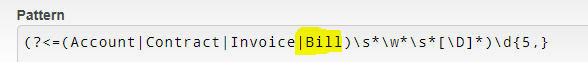# RegeEX how to avoid a word

Hi,
I am trying to use regular expression match.
Account Number/Contract Number/Customer Number/Number.
I am trying to avoid Invoice Number.
The following is not helping to avoid Invoice Number. Can you help?

(?<=Contract No.: |Account #: |Account Number:?|(?! Invoice) Number:)\s?[A-Za-z0-9-.]+[\d-.,]+(?=(.*\n)?)

Thank you,

Please share the text and also highlight the part that you want to extract

By using the following in my regex I am trying to avoid Invoice Number: 12345
(?!Invoice) Number:

(?<=Contract No.: |Account #: |Account Number:?|(?!Invoice) Number:)\s?[A-Za-z0-9-.]+[\d-.,]+(?=(.*\n)?)

It is still matching “Invoice Number: 12345”

Thank you,

Hi, The following is matching

((?i)(?<=Contract No.: |Account #: |Acct\s?#|Acct: |Account:|(ACCT NO.)|Account No.|ACCOUNT NUMBER(:)?)|((?<!Invoice\s)Number:))\s?[A-Za-z0-9-.]+[\d-.,]+(?=(.*\n)?)

Account Number: 123456

giving me “Number: 123456”

How to not match Number: in the above?
That is I need to get only “123456”

Thank you so much.

Hello

Try this:
((?i)(?<=Contract No.: |Account #: |Acct\s?#|Acct: |Account:|(ACCT NO.)|Account No.|ACCOUNT NUMBER(:)?)|((?<!Invoice\sNumber:)))\s?[A-Za-z0-9-.]+[\d-.,]+(?=(.*\n)?)

Cheers

Steve

Hi,

``````(?i)(?<=(Contract|ACCT|ACCOUNT\s*)\s*(No\.|NUMBER|#)?\s*:?\s*)[A-Za-z0-9-.]+[-\d.,]+
``````

Regards,

`(?<=Contract No.: |Account #: |Account Number:?|(?<!\bInvoice )Number:)\s?[A-Za-z0-9-.]+[\d-.,]+(?=(.*\n)?)`

I hope it helps!!

This Regex is nicely organized without repetition. It is fetching No. also from the following data. Can this be fixed? Thanks a lot,

Account No.1234-5678901234

This is working good. Thanks! I am trying to pick the other solution because it looks simpler. Thanks a lot!

Slightly modified to cover all key words and it is not fully applicable.
(?i)(?<=Contract No.: |Account\s?#?:? |Account Number:?|Account No.|Acct:?\s?(NO.)?|(?<!\bInvoice )Number:)\s?[A-Za-z0-9-.]+[\d-.,]+(?=(.*\n)?)

1. Getting “No.” also from data
Account No.1234-5678901234
2. Not capturing
Power Acct: 111-0652.000 1234
3. Not capturing the following for some reason
Account Number 1234567890

Thank you,

1 Like

Thank you @Steven_McKeering

Hi,

If the numeber always starts with numeric character, the following will work. Can you try this?

`(?i)(?<=(Contract|ACCT|ACCOUNT)\s*(No\.|NUMBER|#)?\s*:?\s*)\d[A-Za-z0-9-.]*[-\d.,]+`

Regards,

(?<=(Account|Contract|Invoice)\s*\w*\s*[\D]*)\d{5,}If you need to add additional words for example “Bill”. Then simply add “|Bill” in the first set of brackets (see the bolded text)The invoice number must 5 or more digits in length to match. This can be increased/decreased by adjusting the 5 at the end.

Preview the regex pattern here

Cheers

Steve

Thank you, it does not cover alphabetic.
For example A124-56-89N
ABCD-1234-5678
Appreciate the nicely organized regex.

@Yoichi